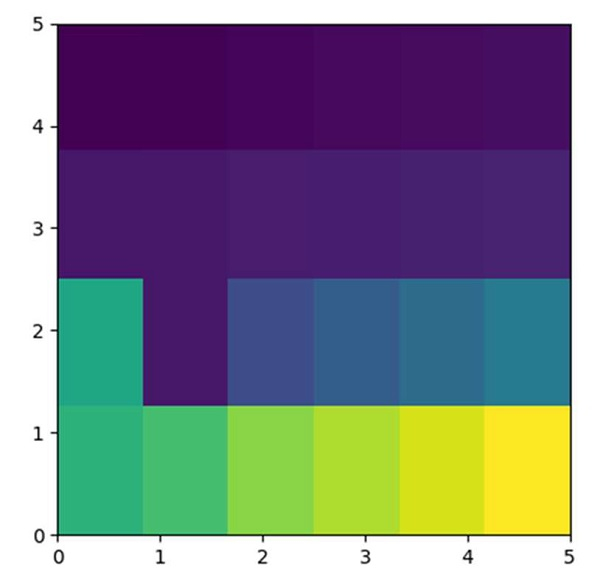# Change values on matplotlib imshow() graph axis

First, we can initialize an array matrix and pass it into the imshow method that can help to get the image for the given matrix.

## Steps

• Create a 2D Array i.e., img.

• Using imshow() method, display the data as an image, i.e., on a 2D regular raster.

• Use plt.show() method to show the figure.

## Example

import matplotlib.pyplot as plt

img = [[1, 2, 4, 5, 6, 7],
[11, 12, 14, 15, 16, 17],
[101, 12, 41, 51, 61, 71],
[111, 121, 141, 151, 161, 171]]

plt.imshow(img, extent=[0, 5, 0, 5])
plt.show()

## Output